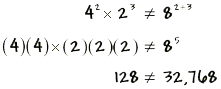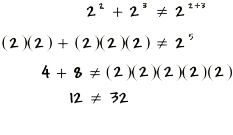Home    |    Teacher    |    Parents    |    Glossary    |    About UsAn exponent tells you how many times the base number is used as a factor. A base of five raised to the second power is called "five squared" and means "five times five." Five raised to the third power is called "five cubed" and means "five times five times five." The base can be any sort of number--a whole number, a decimal number, or a fraction can all be raised to a power.

Here are some simple rules to use with exponents.

1. a1 = a
Any number raised to the power of one equals the number itself.
2. For any number a, except 0, a0 = 1
Any number raised to the power of zero, except zero, equals one.
3. For any numbers a, b, and c,
ab x ac = ab+c

This multiplication rule tells us that we can simply add the exponents when multiplying two powers with the same base.

ALERT! These are mistakes that students often make when dealing with exponents.

Mistake! Do not multiply the base and the exponent. 26 is not equal to 12, it's 64!

Mistake! The multiplication rule only applies to expressions with the same base. Four squared times two cubed is not the same as 8 raised to the power two plus three.Mistake! The multiplication rule applies just to the product, not to the sum of two numbers.Scientific Notation
What happens when you're using a calculator and your answer is too long to fit in the window? Use a calculator to multiply these 2 numbers:

60,000,000,000,000 x 20,000,000,000
You'll discover a short way of writing very long numbers. This is called scientific notation, or E notation on a calculator ("E" stands for "Exponent"). A number written in scientific notation is written as a product of a number between 1 and 10 and a power of 10.

For example, to write 127,680,000 in scientific notation, change the number to a number between 1 and 10 by moving the decimal point 8 places to the left. Then multiply by 10 raised to the power of the number of places you had to move the decimal point--that is, 108:

127,680,000 = 1.2768 x 108
On your calculator window, the base of 10 is not shown; the E means "10 raised to the following power."

Examples
7 x 7 x 7 x 7 = ?    74
2 x 2 x 2 x 2 x 2 x 2 = ?   26
110 =    1
53 =    5 x 5 x 5 = 125

Write the following numbers in scientific notation.
565,000  =  5.65 x 105
7,325,000  =  7.325 x 106
91,247,000,000  =  9.1247 x 1010

 Homework Help | Pre-Algebra | NumbersEmail this page to a friendSearch·  Place value·  Decimal numbers·  Estimating and     rounding·  Adding / subtracting     decimals·  Multiplying decimals·  Dividing decimals·  Percent·  Exponents·  Square roots·  Signed integers·  Adding and     subtracting integers·  Multiplying and     dividing integers·  Properties of integersFirst Glance In Depth Examples WorkoutExponents